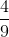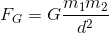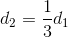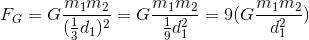# SAT II Physics : Gravitational Force and Weight

## Example Questions

### Example Question #11 : Sat Subject Test In Physics

If the distance between two objects is reduced by two-thirds, how will the gravitational force between the objects be affected?

Increases by a factor ofDecreases by a factor ofIncreases by a factor ofDecreases by a factor ofIncreases by a factor ofIncreases by a factor ofExplanation:

According to Newton's law of universal gravitation, the gravitational force between two objects is inversely proportional to the square of the distance between them.If the distance is reduced by two-thirds, then the final distance is equal to one-third the original distance.Using this term in the equation will show that the force increases by a factor of nine.